To catch and reverse a quantum jump...

And what about the theory? German VersionI think most physicists, even though they may profess faithful belief in the Copenhagen interpretation, still share with me a disreputable, materialistic prejudice that stones and trees cannot be either more - or less - real than the atoms of which they are composed. And, if it is meaningless to ask what an individual moment is doing, can it be any more meaningful to ask what their sum is doing? E.T. Jaynes, Survey of the present status of neoclassical radiation theory.

The quote from E.T. Jaynes is perhaps not as well known as Schrödinger's "In the first place it is fair to state that we are not experimenting with single particles, any more than we can raise Ichthyosauria in the zoo." (Are There Quantum Jumps? Part I, The British Journal for the Philosophy of Science, 3, (1952), 109-123 [B 12]), and of course Schrödinger's cat is even better known. The problem is always the same: does quantum theory (QT) describe the behavior of an ensemble or individual particles? And the question arises whether there is an "objective randomess of the QT" (quantum indeterminism), or a deterministic "development of the individual" (cat, dinosaur or atom) runs continuously, or only "jumps" to a value upon observation. Not to mention the multitude of interpretations of the QT with which one tries to sweep these contradictions under the rug.
Fortunately, there are experiments! Yes - you can experiment with individual atoms, at least since Dehmelt, but you can now also produce "artificial atoms" that can be "manipulated macroscopically". In other words, there is no longer any sharp boundary between quantum physics and classical physics (if there ever was).
One of these more recent experiments is Minev's "To catch and reverse a quantum jump mid-flight". It is based on Dehmelt's idea of ​​indirectly observing the behavior of the individual: An artificial atom with three levels (V-scheme) is stimulated to two transitions simultaneously. One of them fluoresces (= bright), the other is metastable (= dark). If the bright signal fails to appear, this is an indication that the atom is in the "dark state" or is just making its way there. In Dehmelt's time, the resulting "telegraph signal" was interpreted as evidence of instantaneous quantum jumps. Minev's experiment shows (once more) that the transition to the dark state takes place continuously in a finite time, and can even be reversed. Highest level of experimental art!
The trick is to find the right moment at which the individual, apparently by chance, decides to become metastable, while relying on the ensemble (i.e. millions of observations).
Is there a theory about it? Yes, even several. One of them is called Quantum Trajectory Theory (QTT): The "objective randomness of quantum physics" is shifted to the ensemble and, with the help of a "stochastic Schrödinger equation" and "super operators", "quantum trajectories" are constructed, which are triggered by "omniscient observers" (Greetings from Monte Carlo). If the lot falls on a transition to the dark state, this transition is described with the completely normal (deterministic and linear) Schrödinger equation.

However, another problem arises from this: the linear Schrödinger equation always leads to linear differential equations, i.e. to (a sum of) exponential functions as a solution (see Weisskopf-Wigner). While the temporal evolution of the transition measured by Minev et al. is described by a tanh-function (curve fit), which is typical for a non-linear process.

We therefore want to take a closer look at whether it is really possible to derive a non-linear result from a linear theory (QTT).

From arXiv:1803.00545v3 [quant-ph] 12 Feb 2019, Supplement page 4: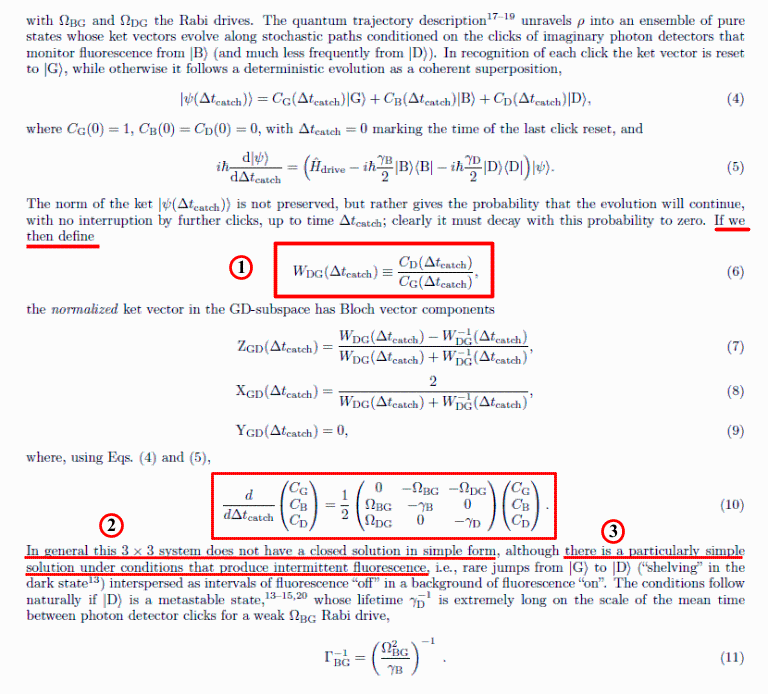Supplement page 5: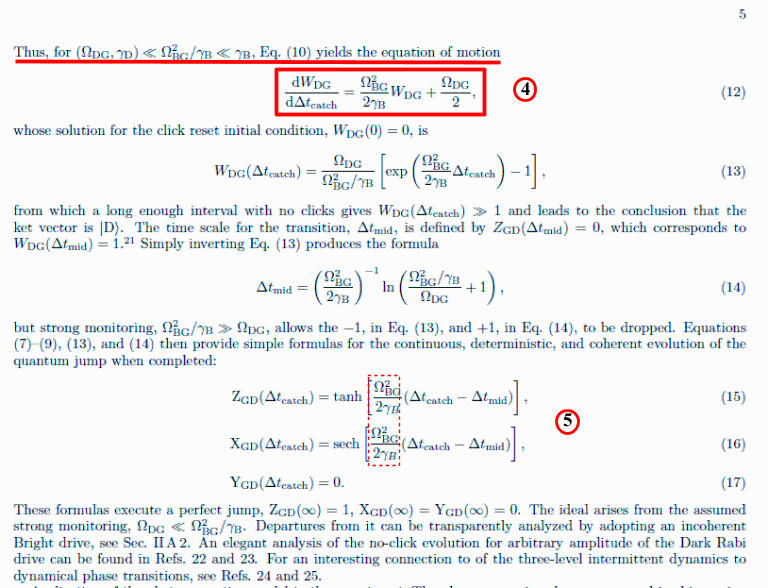From a purely mathematical point of view, the procedure for deriving (5) can be divided into an "exact solution (3x3-system)" and an "approximate solution (2x2-system)":

Exact solution (3x3-system)
(The labels on the far right, starting with (2.1), are Maple-worksheet labels)

To (1) "if we then define":

With the definition(2.1)

the "usual notation of inversion" (squares of the amplitudes = densities)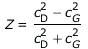(2.2)

becomes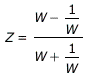(2.3)

One wonders what this definition is good for (also for the X and Y components of the Bloch vector).

To (2) "In general this 3 x 3 system does not have a closed solution in simple form":

State of the atom with G = ground, B = bright, D = dark: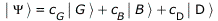(2.4)

So a superposition of 3 states with the amplitudes c

Equation (5) (Supplement page 4) leads to the matrix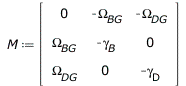(2.5)

respectively the  3x3-DE-sytem  for the amplitudes c.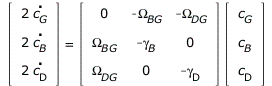(2.6)

or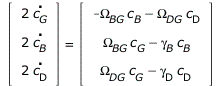(2.7)

Note:
1. An excited state (B or D) that "spontaneously decays" (γ) does not change the ground state G in this representation (decays to nowhere)!
2. This is a homogeneous linear DE-system with constant coefficients. The solutions of such DE-systems always consist of linear combinations of exponential functions (possibly in complex form), but can also have "no simple form" if, for example, a cubic equation has to be solved for the eigenvalues.
In this case the real (in any case real) eigenvalue is e.g.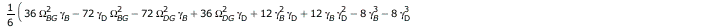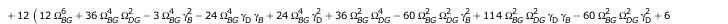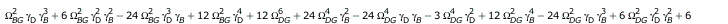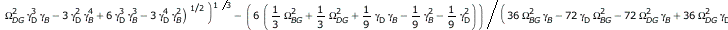(2.9)

So there is a closed solution (there is always a numerical one), but with Maple's solution algorithm it is so extensive that it has "no simple form" (the output comprises more than 1 million characters :).

But you can represent them graphically, e.g. the

Squares of the magnitudes of the amplitudes c:

Red: ground state (G), green: dark state (D), blue: bright state (B), values ​​of the four parameters in MHz, animations with 1 frame per second.

1. Order of magnitude of the numbers as in Minev et al., the decay rate of the dark state is changed.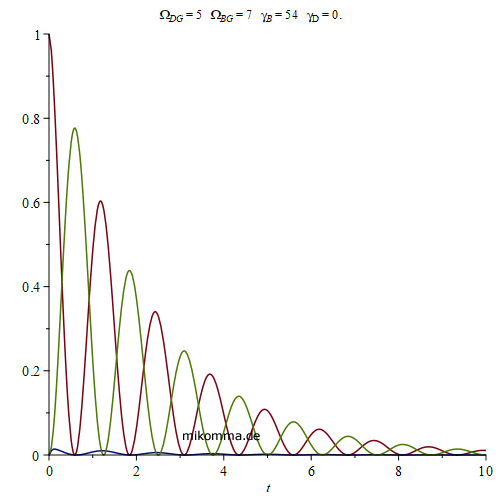The amplitude of the bright state is practically zero!

2. As 1., but with a small decay rate of the bright state, which is now visible.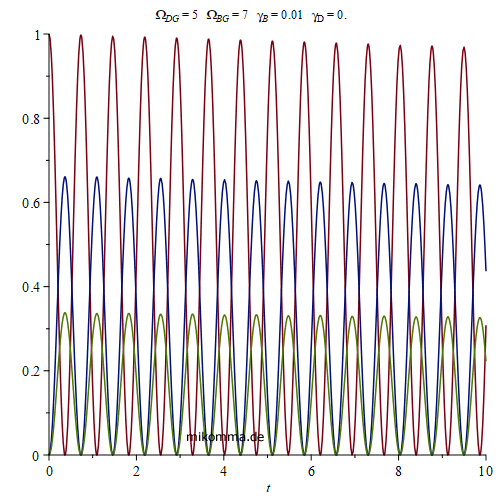3. The Rabi frequency of the DG transition is changed. "Damped oscillation" furthermore...4. The inversion Z (light blue) is also shown. The Rabi frequency of the BG transition is changed. Transition from Z periodic, to Z aperiodic.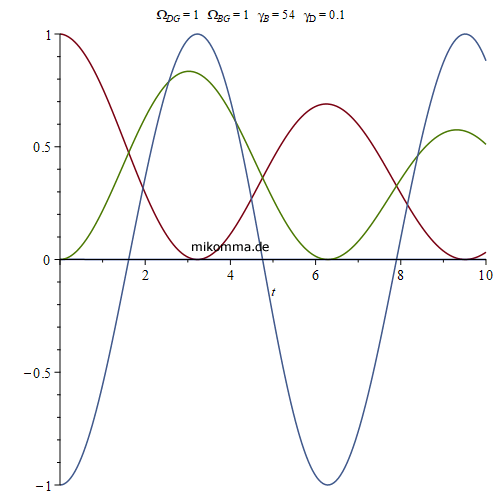5. Amplitude squares of G and D increased by a factor of 100. ΩDG is reduced. Z becomes aperiodic and approaches a "tanh-curve" because the zeros of cG und cD "dissapear" (Rabi-frequency  0), and approaches cD/cG~exp(k*t) (cf. short-lived mother, long-lived daughter).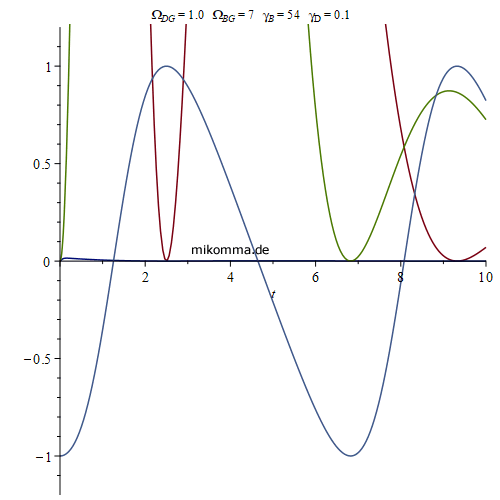But you can also just insert numbers to see the simple form of the solution, e.g .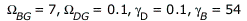(2.10)

results in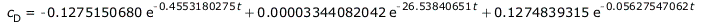(2.11)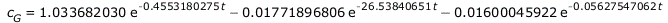(2.12)

thus the mentioned linear combination of exponential functions (here with real eigenvalues).

Problematic:
- A superposition of three states is assumed (not normalized). All three states decay exponentially. In the end, the atom is not in any of the three states! Nevertheless, the inversion approaches the value "1 = 0/0" (as in the case of radioactive decay with a short-lived mother and long-lived daughter). In other words the inversion Z is a percentage value (which automatically renormalizes the state), but says little about the (absolute) state of the atom!
- The solution of the 3x3 system is discussed in detail in an article by Porrati and Putterman 1989 (see below), but only the article by Porrati and Putterman 1987 is cited.

Approximate solution (2x2-System)

To (3)  "although there is a particularly simple solution under conditions that produce intermittent fluorescence"

The solution of the 3x3 system, which is known in principle, is not used. Instead, an approximation is introduced (which can only be found in the dissertation): The change in the bright state (B) over time is set to zero, which means that the bright state can be eliminated from the 3x3 system, and a homogeneous linear 2x2- DE-system with constant coefficients results (in classical mechanics one thinks of "damped coupled oscillations", in QO of "Rabi oscillations"):(3.1)

The solution of this DE-system is in a closed form: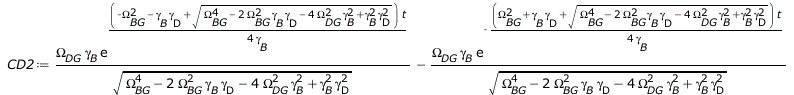(3.3)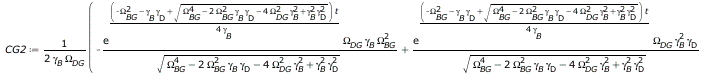(3.4)

In other words, an exact and manageable solution that can of course also be calculated without CAS.

Instead of animations, here are just a few examples of how you can set the parameters with Maple with sliders, e.g. for the amplitudes :

CG and CD  (red and blue)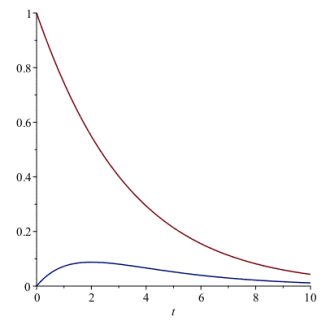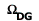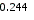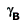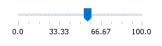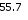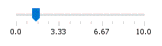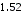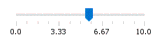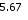or the components Z und X of the Bloch-vector

(Z red und X blue):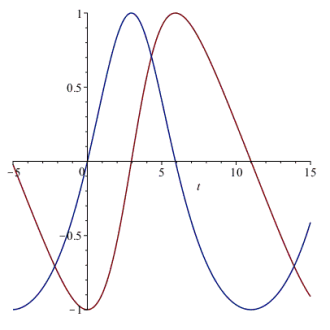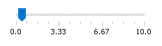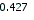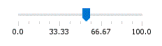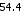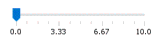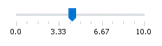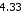With a zoom (changed ranges of the slider)
you can even approach a "tanh-curve" ...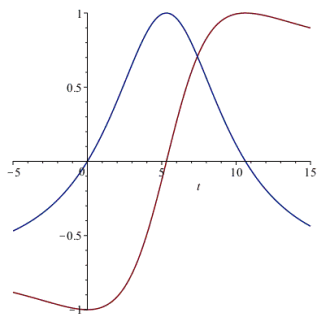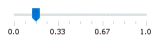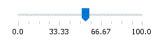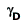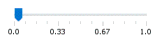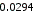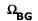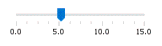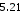With the exact solution of the 2x2 system, it is easy to investigate how the change in the four parameters affects the Rabi-oscillations of a 2-level system. But even this simplified solution (particularly simple solution!) is not used to describe the GD-transition directly. Instead, with the definition (1) for W and the 2x2 system, an equation of motion for W is set up:

To (4) equation of motion for W

The 2x2 system (see above, (3.1)) is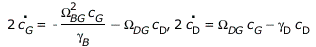(3.5)

With the definition (1) for W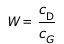(3.7)

the equation of motion for W is obtained: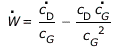(3.8)

Insertion of the time derivatives of the amplitudes c from the 2x2 system gives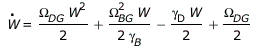(3.12)

The solution W(t) of this DE is a tan-function, which does not become a tanh-function even for negative radicands of the root: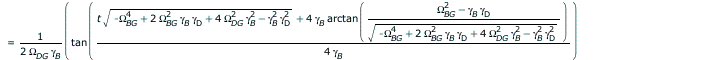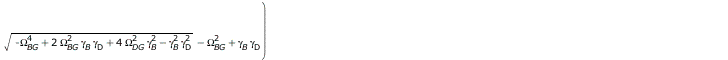(3.13)

But this exact solution is not used either (for good reason: it is "unphysical"). Instead, the equation of motion for W is replaced by this approximationin which, however, beyond the approximation mentioned above, the quadratic term in W is also missing, which would only make sense for W < 1. With these simplifications (and the neglect of a summand -1) one then obtains an exponential function for W, and finally

To (5)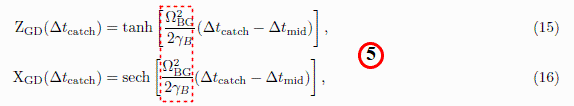These equations are then used to fit the experimental data.

The main problem with the whole derivation is the result itself: The time development of the transition from the ground state (G) to the dark state (D), i.e. the observed "deterministic quantum jump", does not depend on the parameters (decay constant and Rabi frequency) of the dark state , but is determined exclusively by the parameters of the bright state (B). As a reminder: The amplitude of the bright state is theoretically almost zero "for all times"!

In addition, the following measurement presented in the article itself contradicts the theory that has been put forward:

Quoting from Nature 570, 200–204 (2019). https://doi.org/10.1038/s41586-019-1287-z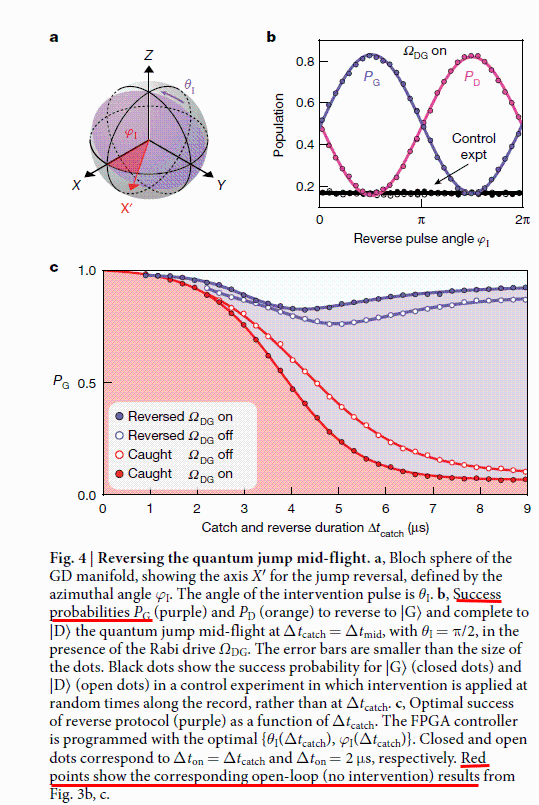Note 1: "b, Success probabilities PG (purple) and PD (orange) to reverse to |G〉 and complete to |D〉 the quantum jump mid-flight at Δtcatch = Δtmid, with θI = π/2, in the presence of the Rabi drive ΩDG."

The phrase "probability of success to reverse the transition (or not)" probably means the density (population) of one or the other state (G or D). This means that PG = CG2 ~ (1-tanh(kt/2))/2 was measured (Fig. c, time-shifted), which contradicts the linear approach of the "whole theory".

Note 2: With CG2 ~ (1-tanh(kt/2))/2 follows Z~tanh(kt/2), which can be shown most easily if one calculates with densities instead of amplitudes, see e.g. . For the amplitudes one would then have differential equations of the form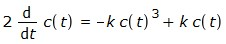i.e. non-linear differential equations that cannot be represented with the QTT (and the linear Schrödinger equation used there).

Summary

1. With the QTT, a 3x3 DE-system is set up for the amplitudes, which has an exact but unwieldy solution (cubic equation).

2. The solution of the 3x3 system would provide a tanh-like function for the inversion Z, ie "confirm" the measurement (as a curve fit). The amplitudes and densities would be "decay curves" (and not tanh-curves).

3. The solution of the 3x3 system, which is known in principle, is not used, but a 2x2 system is set up in which the bright state is set constant.

4. In the 2 level system described in this way, an equation of motion is set up for the quotient W of the amplitudes c, which is "solved" with further approximations (the exact solution would be "unphysical").

5. The result of this procedure (this chain of approximations and simplifications) is a tanh function with which one can make a curve fit for the inversion Z, with the decay rates serving as "free parameters" (which are not measured). In particular, the dark state does not appear in this result, which is intended to describe the transition to the dark state, but the time development of the transition is described exclusively by the parameters of the light state.

6. The measurement of the density of states
PG shows a tanh-curve, in contradiction to the amplitudes / densities of the established theory.

7. A tanh-function of the densities would also lead to a tanh-function of the inversion, but cannot be derived from the linear Schrödinger equation, not even with QTT.

8. A consistent treatment of atomic transitions can be found in Ensemble - Individuum, or in Über die spontane Emission von Photonen (quote with the symbols from there):

"The change of the ground state ρgg is proportional to the square of the dipole moment ρgg (1-ρgg) = radiated power.

Putting this physical fact in the foreground instead of assuming an "exponential decay" as the solution, one obtains the differential equation with the proportionality constant k:also known as the logistic differential equation. It has the "standardized" solution (ρgg(0)=1/2):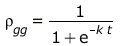=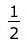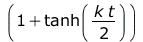also known as the logistic function, or cumulative logistic distribution."

The question now is how, of all things, a series of approximations can be used to arrive at a tanh-solution that was already known to the neoclassic, without citing the neoclassic (e.g. E.T. Jaynes).

9. The decay constants are not determined directly in the experiment, but serve as "free parameters" that are calculated / adapted.

10. In the transition to the dark state, only the bright state occurs in the time constant. (Only the amplitude of W is co-determined by the rabi frequency of the dark state.)

11. A value for the dark state decay constant is nowhere to be found in the article. It is eliminated by the assumption γD → 0.

Sources:

Minev et al.:
- Dissertation: Catching and Reversing a Quantum Jump Mid-Flight, Zlatko Kristev Minev 2018, https://arxiv.org/pdf/1902.10355.pdf
- arXiv: "To catch and reverse a quantum jump mid-flight":
arXiv:1803.00545v3 [quant-ph] 12 Feb 2019, https://arxiv.org/abs/1803.00545
- Nature 570, 200–204 (2019). https://doi.org/10.1038/s41586-019-1287-z,
https://www.nature.com/articles/s41586-019-1287-z

Porrati et al.:
- M. Porrati, S. Putterman, Phys. Rev. A36, 929 (1987)
Wave-function collapse due to null measurements: the origin of intermittent atomic fluorescence.

- M. Porrati, S. Putterman, Phys. Rev. A39, 3010 (1989)
Coherent intermittency in the resonant fluorescence of a multilevel atom.

© Juni 2021, Dr. Michael Komma (VGWORT)